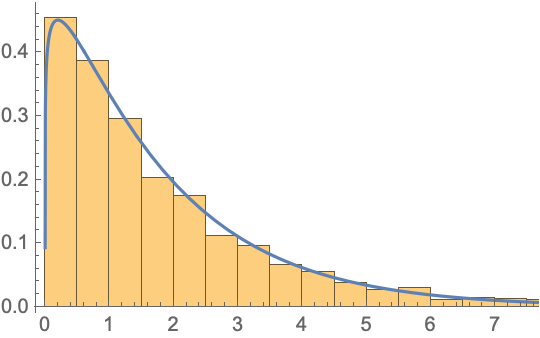#Function Repository Resource:

# SerialRandomnessTest

Conduct an empirically derived test that assesses randomness using the frequencies of serial combinations of 0s and 1s

Contributed by: Emmy/Noah Blumenthal
noahb320@gmail.com
emmyb320@bu.edu
 ResourceFunction["SerialRandomnessTest"][sequence] conducts an empirically derived test using the frequencies of serial combinations of 0s and 1s in sequence and returns an associated p-value. ResourceFunction["SerialRandomnessTest"][sequence,"properties"] conducts an empirically derived test and returns the specified property.

## Details and Options

Properties include:
 "TestStatistic" returns the test statistic "PValue" returns the p-value associated with the test
The test statistic is generated by creating a chi-square-like statistic that compares the frequencies of subsequences of 0s and 1s of length two to the expected frequencies.
The test works only for sequences of 0s and 1s.
Results are generally invalid for sequence lengths short than 100.

## Examples

### Basic Examples

Generate a sequence of random integers and apply a runs-based test:

 In:=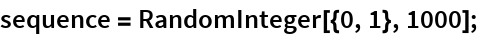Visualize the sequence:

 In:=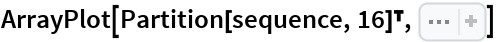Out=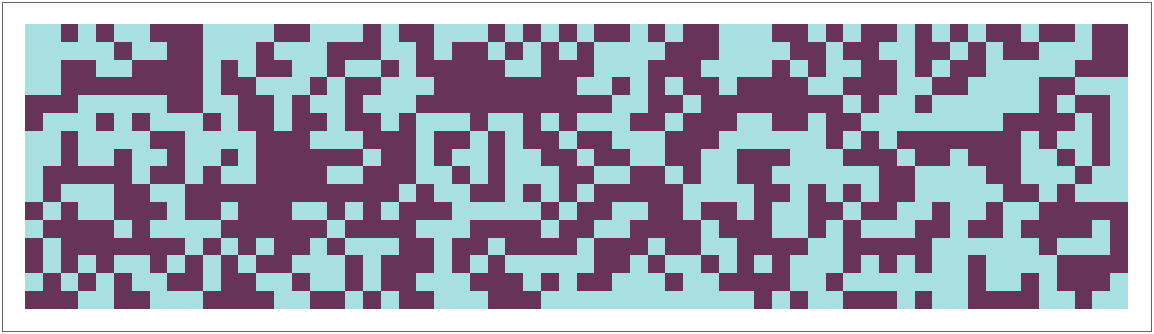In:=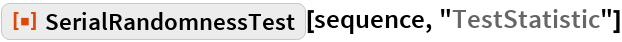Out=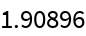In:=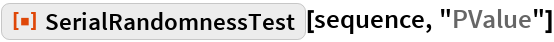Out=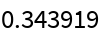### Applications

Reject a non-random sequence: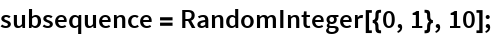In:=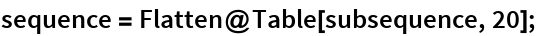In:=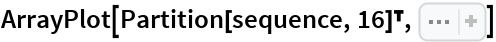Out=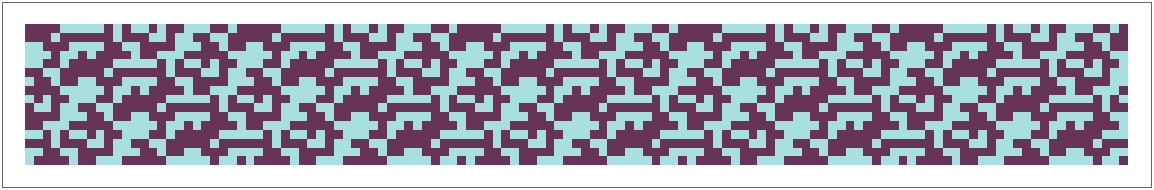In:=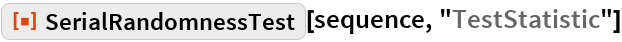Out=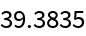In:=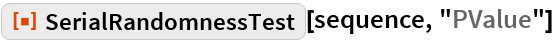Out=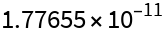Test the randomness of rule 30:

 In:=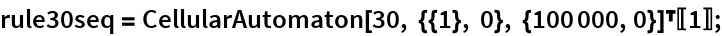In:=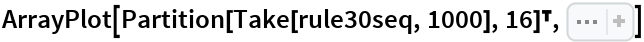Out=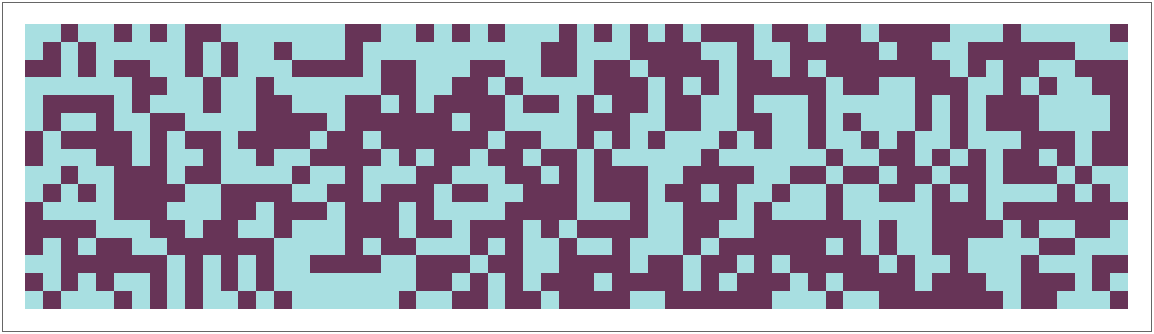In:=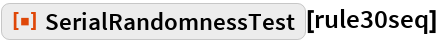Out=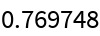### Possible Issues

SerialRandomnessTest requires sequences of length 100 or more:

 In:=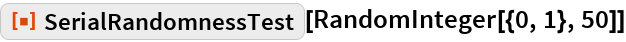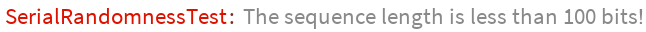### Neat Examples

Visualize the sampling distribution of the test statistic:

 In:=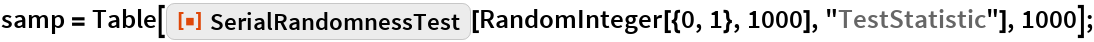In:=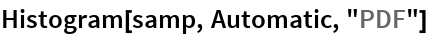Out=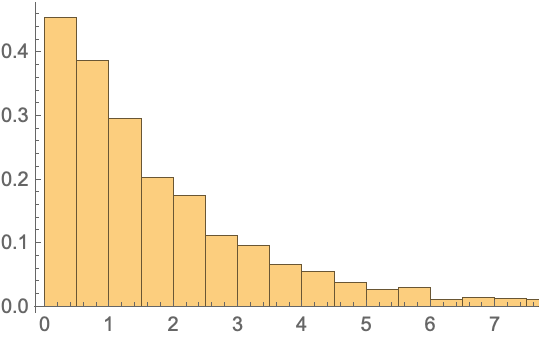In:=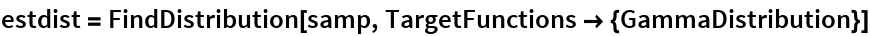Out=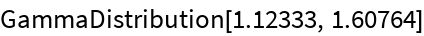In:=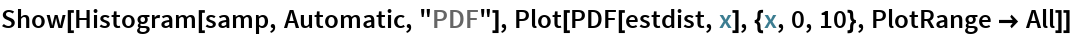Out=